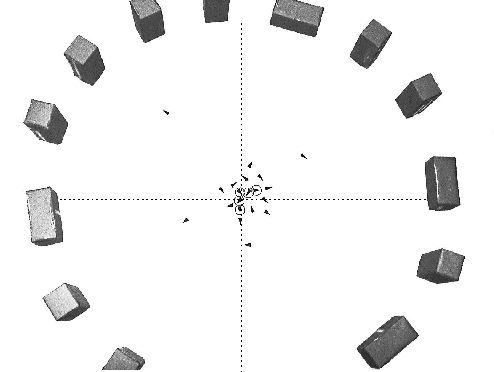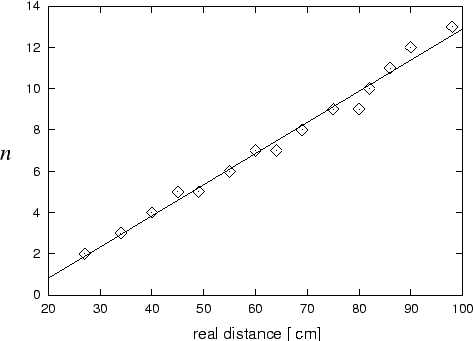Next: 7.4 Data outside the Up: 7.3 Results Previous: 7.3.4 Goal-directed movements

## 7.3.5 Mental transformation

Using mental transformation, the robot could determine whether it was standing in the middle of the circle (figure 7.13), and it could determine the distance to the obstacle in front (figure 7.14).

On the first test, figure 7.13 shows which locations were classified as being in the center and which not. Among the positions that were classified as center, the maximum distance to the center was 10 cm.

On the second test, the number of predicted steps toward the obstacles scaled with the real distance (figure 7.14). A line was fitted to the data. In all trials, the deviation from the line was less then one step. From the slope of the line the speed of the simulated movement can be estimated. The slope is 6.6±0.2 cm/step and corresponds to a speed of 33±1 mm/sec (the actual speed for the given velocity command was 33.5 mm/sec: 40mm/sec divided by a conversion factor for the wheels).Next: 7.4 Data outside the Up: 7.3 Results Previous: 7.3.4 Goal-directed movements
Heiko Hoffmann
2005-03-22